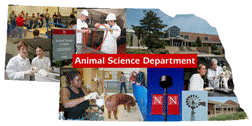## Animal Science Department## Faculty Papers and Publications in Animal Science

#### Date of this Version

11-3-2004

Published in J. Anim. Sci. 2004. 82:3421–3429. Copyright American Society of Animal Science. Used by permission.

#### Abstract

Quantitative trait loci for reproductive traits in a three-generation resource population of a cross between low-indexing pigs from a control line and high-indexing pigs from a line selected 10 generations for increased index of ovulation rate and embryonic survival are reported. Phenotypic data were collected in F2 females for birth weight (BWT, n = 428), weaning weight (WWT, n = 405), age at puberty (AP, n = 295), ovulation rate (OR, n = 423), number of fully formed pigs (FF, n = 370), number of pigs born alive (NBA, n = 370), number of mummified pigs (MUM, n = 370), and number of stillborn pigs (NSB, n = 370). Grandparent, F1, and F2 animals were genotyped for 151 microsatellite markers. Sixteen putative QTL (P < 0.10) for reproductive traits were identified in previous analyses of these data with single QTL line-cross models. Data were reanalyzed with multiple QTL models, including imprinting effects. Data also were analyzed with half- sib models. Permutation was used to establish genomewide significance levels (α = 0.01, 0.05, and 0.10). Thirty-one putative QTL for reproductive traits and two QTL for birth weight were identified (P < 0.10). One Mendelian QTL for FF (P < 0.05), one for NBA (P < 0.05), three for NSB (P < 0.05), three for NN (P < 0.05), seven for AP (P < 0.10), five for MUM (P < 0.10), and one for BWT (P < 0.10) were found. Partial imprinting of QTL affecting OR (P < 0.01), BWT (P < 0.05), and MUM (P < 0.05) was detected. There were four paternally expressed QTL for NN (P < 0.10) and one each for AP (P < 0.05) and MUM (P < 0.10). Maternally expressed QTL affecting NSB (P < 0.10), NN (P < 0.10), and MUM (P < 0.10) were detected. No QTL were detected with half-sib analyses. Multiple QTL models with imprinting effects are more appropriate for analyzing F2 data than single Mendelian QTL line-cross models.

COinS